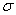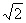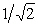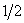Name:    Chapter 9: Sampling Distributions

Multiple Choice
Identify the choice that best completes the statement or answers the question.

1.

Following a dramatic drop of 500 points in the Dow Jones Industrial Average in September 1998, a poll conducted for the Associated Press found that 92% of those polled said that a year from now their family financial situation will be as good as it is today or better.  The number 92% is a
 a. Statistic b. Sample c. Parameter d. Population e. None of the above.

2.

In a large population, 46% of the households own VCR’s.  A simple random sample of 100 households is to be contacted and the sample proportion computed.  The mean of the sampling distribution of the sample proportion is
 a. 46 b. 0.46 c. About 0.46, but not exactly 0.46 d. 0.00248 e. The answer cannot be computed from the information given

3.

If a population has a standard deviation, then the standard deviation of the mean of 100 randomly selected items from this population is
 a.b. 100c./10 d./100 e. 0.1

4.

A statistic is said to be unbiased if
 a. The survey used to obtain the statistic was designed so as to avoid even the hint of racial or sexual prejudice b. The mean of its sampling distribution is equal to the true value of the parameter being estimated c. Both the person who calculated the statistic and the subjects whose responses make up the statistic were truthful d. It is used for honest purposes only e. None of the above.

5.

The number of undergraduates at Johns Hopkins University is approximately 2000, while the number at Ohio State University is approximately 40,000.  At both schools a simple random sample of about 3% of the undergraduates is taken.  Which of the following is the best conclusion?
 a. The sample from Johns Hopkins has less sampling variability than that from Ohio State. b. The sample from Johns Hopkins has more sampling variability than that from Ohio State. c. The sample from Johns Hopkins has almost the same sampling variability as that from Ohio State. d. It is impossible to make any statement about the sampling variability of the two samples since the students surveyed were different. e. None of the above.

6.

In a large population, 46% of the households own VCRs.  A simple random sample of 100 households is to be contacted and the sample proportion computed.  What is the standard deviation of the sampling distribution of the sample proportion?
 a. 46 b. 0.46 c. 0.00248 d. 0.005 e. None of the above.

7.

In a large population of adults, the mean IQ is 112 with a standard deviation of 20.  Suppose 200 adults are randomly selected for a market research campaign.  The distribution of the sample mean IQ is
 a. Exactly normal, mean 112, standard deviation 20. b. Approximately normal, mean 112, standard deviation 0.1. c. Approximately normal, mean 112, standard deviation 1.414. d. Approximately normal, mean 112, standard deviation 20. e. Exactly normal, mean 112, standard deviation 1.414.

8.

Suppose we are planning on taking an SRS from a population.  If we double the sample size, thenwill be multiplied by:
 a.b.c. 2 d.e. 4

A factory produces plate glass with a mean thickness of 4 millimeters and a standard deviation of 1.1 millimeters. A simple random sample of 100 sheets of glass is to be measured, and the sample mean thickness of the 100 sheetsis to be computed.

9.

We know the random variablehas approximately a normal distribution because of
 a. the law of large numbers. b. the central limit theorem. c. the law of proportions. d. the fact that probability is the long run proportion of times an event occurs.

10.

The probability that the average thicknessof the 100 sheets of glass is less than 4.1 millimeters is approximately
 a. 0.8186. c. 0.1814. b. 0.3183. d. 0.6817.